Courses

# Systematic sampling (Part - 1), CSIR-NET Mathematical Sciences Mathematics Notes | EduRev

## Mathematics : Systematic sampling (Part - 1), CSIR-NET Mathematical Sciences Mathematics Notes | EduRev

The document Systematic sampling (Part - 1), CSIR-NET Mathematical Sciences Mathematics Notes | EduRev is a part of the Mathematics Course Mathematics for IIT JAM, CSIR NET, UGC NET.
All you need of Mathematics at this link: Mathematics

The systematic sampling technique is operationally more convenient than the simple random sampling.  It also ensures  at the same time that each  unit has equal probability of inclusion in the sample. In this method of  sampling, the first unit is selected  with the help of random numbers  and the remaining units are selected automatically according to a predetermined pattern.  This method is known as systematic  sampling.

Suppose the N units in the population are numbered 1 to N in some order. Suppose further that  N is expressible as a product of two  integers n and k, so that N = nK.

To draw a sample of size n,

- select a random number between 1 and  k.
- Suppose it is  i.
- Select the first unit whose serial number is  i.
- Select every kth  unit after ith unit.
- Sample will contain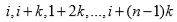serial number units.

So first unit is selected at random and other units are selected systematically. This systematic sample is called  kth  systematic sample  and k is termed as sampling interval.  This is also known as linear systematic sampling. The observations in the systematic sampling are arranged  as in the following table:

 Systematic sample number 1 2 3 ... i ... k Sample composition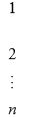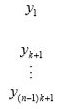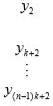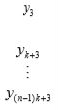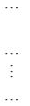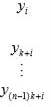...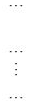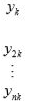Probability 1/k 1/k 1/k ... 1/k ... 1/k Sample mean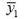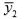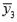...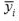...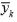Example: Let  N = 50 and  n = 5. So k = 10. Suppose first selected number between 1 and 10 is 3. Then systematic sample consists of  units with following serial number 3, 13, 23, 33, 43.

Systematic sampling in two dimensions:

Assume that the units in a population are arranged in the form of ml rows and each row contains nk units. A sample of size mn is required. Then

- select a pair of random numbers (i, j ) such that i ≤ l and j ≤ k .

- Select the (i, j )th unit, i.e., j th unit in i th row as the first unit.

- Then the rows to be selected  are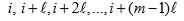and columns to be selected are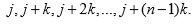- The points at which the m selected rows and  n selected columns intersect determine the position of  mn selected units in the sample.

Such a sample is called an aligned sample.

Alternative approach to select the sample is

- independently select  n random integers i1 ,i2 , ..., isuch that  each of them is less than or equal to ℓ.
- Independently select m random integers j,j2 , ..., jm such  that each of them is less than  or equal to k .
- The units selected in the sample  will have following coordinates: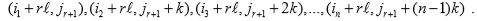Such a sample is called an unaligned sample.

Under certain conditions, an unaligned sample is often superior to an aligned sample as well as a stratified random sample.

1. It is easier to draw a sample and often easier to execute it without  mistakes. This is  more advantageous  when the drawing is done in fields and offices as there may be substantial  saving in time.

2. The cost is low and the selection of  units is simple. Much  less training is needed for surveyors to collect  units through systematic sampling .

3. The systematic sample is spread  more evenly over the population. So no large part will fail to be  represented in the sample. The sample is evenly spread and cross section is better. Systematic sampling fails in case of  too many blanks.

Relation to the cluster sampling

The systematic sample can be viewed from the cluster sampling point of view.  With n = nk , there are  k possible systematic samples. The same  population can be viewed as if divided into k large sampling units, each of which contains n of the original  units. The operation of  choosing a systematic sample is  equivalent to choosing one of the  large sampling unit at random which constitutes the whole sample. A systematic sample is thus a simple random sample of one cluster unit from a population of k cluster units.

Estimation of population mean : When N = nk:

Let yij : observation on the unit bearing the serial number i + ( j− 1)k in the population,

i = 1, 2, ...,k , j = 1, 2, ...,n.
Suppose the drawn random number is i ≤k.
Sample consists of  i th column (in earlier table).
Consider the sample mean given by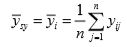as an estimator of the population mean given by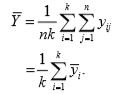Probability of selecting  ith column as systematic sample = 1/k

So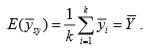Thus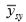is an unbiased  estimator of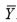.

Further,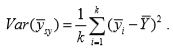Consider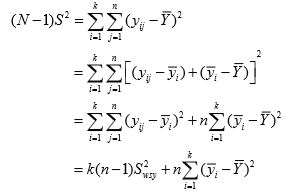where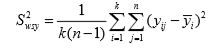is the variation among the units that lie  within the same systematic sample . Thus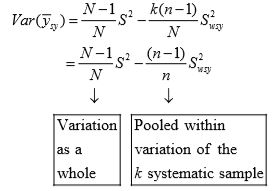with  N = nk . This expression indicates  that when the within variation is  large, then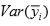becomes  smaller.  Thus higher heterogeneity  makes the estimator more efficient and  higher heterogeneity is well  expected in systematic sample.

Alternative form of variance: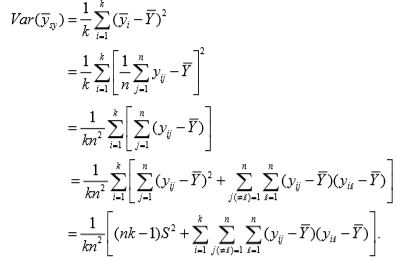The intraclass correlation between the pairs of units that are in the same systematic sample is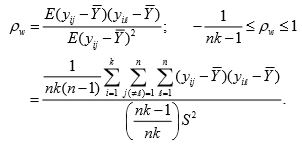So substituting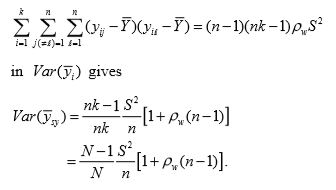Comparison with SRSWOR:

For a SRSWOR sample of size n ,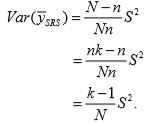Since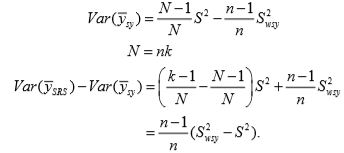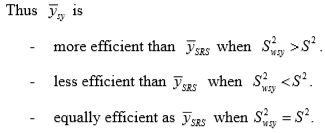Also, the relative efficiency of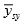relative to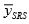is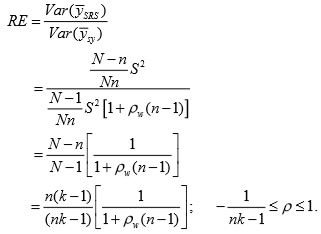Thus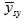is

- more efficient than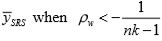- less efficient than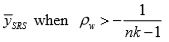- equally efficient as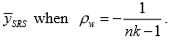Comparison with stratified sampling: The systematic sample can also be viewed as if arising as a stratified sample.  If population of N = nk units is divided into n strata and suppose one unit is randomly drawn  from each of the strata. Then we get a stratified  sample of size n . In doing so, just   consider each row of the following arrangement as a stratum.

 Systematic sample number 1 2 3 ... i ... k Sample composition...Probability 1/k 1/k 1/k ... 1/k ... 1/k Sample mean......Recall that in case of stratified sampling with  k strata, the stratum mean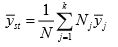is an unbiased estimator of population mean.

Considering the set up of stratified sample in the set up of systematic sample, we have
- Number of strata = n
- Size of strata = k (row size)
- Sample size to be drawn from each stratum = 1

and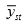becomes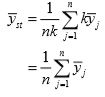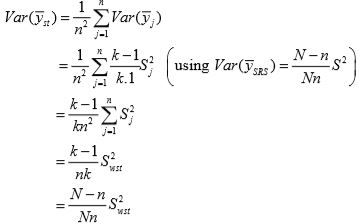where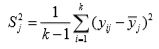is the mean sum of squares of units in the jth stratum.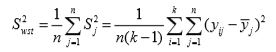is the mean sum of squares within strata (or rows).
The variance of systematic sample mean is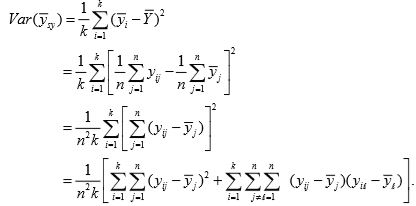Now we simplify and express this expression in terms of intraclass correlation coefficient.  The intraclass correlation coefficient between the pairs  of deviations of units which lie along  the same row measured from their stratum means is defined as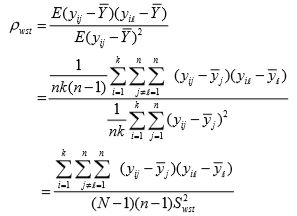So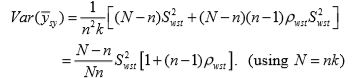Thus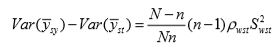and the relative efficiency of systematic  sampling relative to equivalent stratified sampling is given by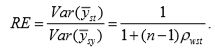So the systematic sampling is
- more efficient than the corresponding equivalent stratified   sample when ρwst > 0 .
- less efficient than the corresponding equivalent stratified   sample when ρwst < 0
- equally  efficient than the corresponding equivalent stratified   sample when ρwst = 0.

Offer running on EduRev: Apply code STAYHOME200 to get INR 200 off on our premium plan EduRev Infinity!

197 docs

,

,

,

,

,

,

,

,

,

,

,

,

,

,

,

,

,

,

,

,

,

,

,

,

;